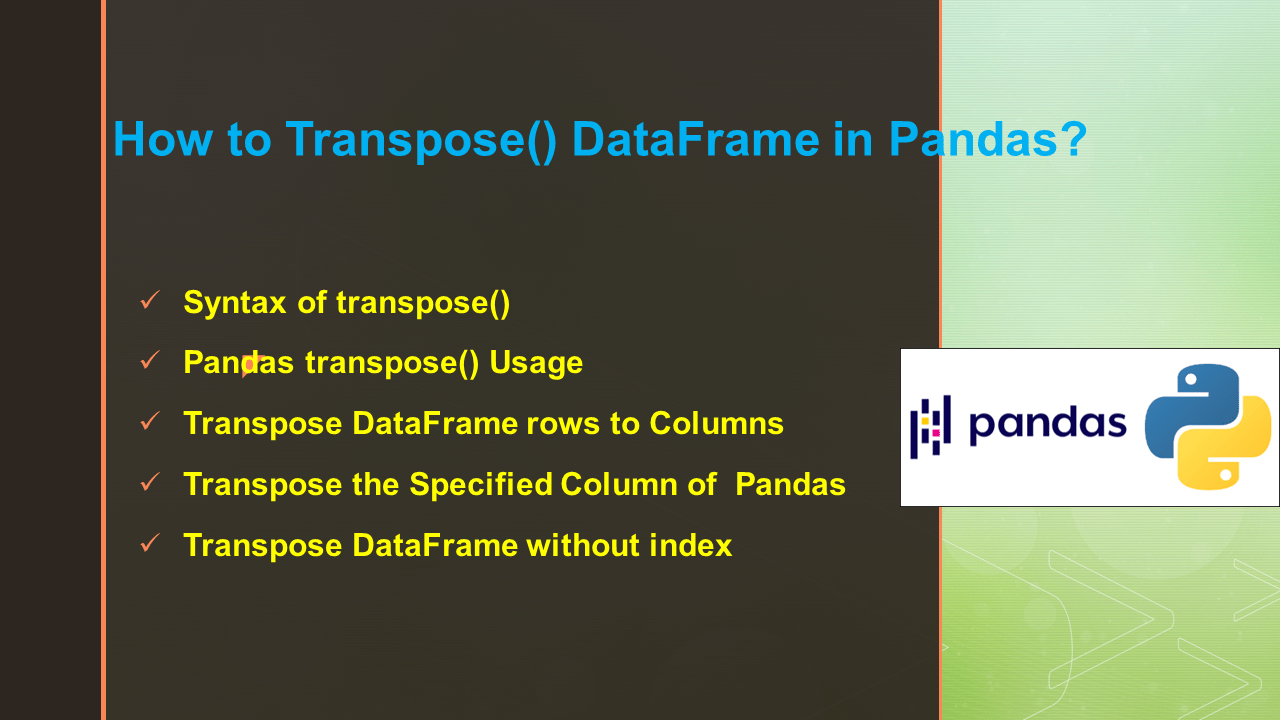# How to Transpose() DataFrame in Pandas?

• Post author:
• Post category:Pandas

Pandas `transpose()` function is used to transpose rows(indices) into columns and columns into rows in a given DataFrame. It returns transposed DataFrame by converting rows of the original DataFrame as columns and vice versa.

In this article, I will explain the concept of `Pandas transpose()` function and using this syntax and parameters how we can transpose the given DataFrame in several ways.

## 1. Quick Examples of Transpose DataFrame

If you are in hurry, below are some quick examples of how to transpose DataFrame.

``````
# Below are some quick examples.

# Example 1: Transpose the rows as columns
transposed_df = df.transpose()

# Example 2: Transpose single column of DataFrame
technologies= {'Fee' :[22000,25000,23000,24000,26000]}
df = pd.DataFrame(technologies)
transposed_df = df.transpose()

# Example 3: Transpose DataFrame without index
transposed_df = df.set_index('Courses').transpose()

# Example 4: Transpose the DataFrame
transposed_df = df.transpose()
# Check the dtype of transposed DataFrame
print(transposed_df.dtypes)
``````

## 2. Syntax of transpose()

Below is the syntax of Pandas transpose()

``````
# Syntax of transpose()
DataFrame.transpose(*args, **kwargs)
``````

### 2.1 Parameters of transpose()

Following are the parameters of transpose() function.

• `copy:` If copy = True, then the underlying data is copied. Otherwise, by default, no copy is made, if possible.
• `*args, **kwargs:` Both are additional keyword.

### 2.2 Return Value

It returns transposed DataFrame.

## 3. Pandas transpose() Usage

Pandas transpose() function is used to interchange the axes of a DataFrame, in other words converting columns to rows and rows to columns. In some situations we want to interchange the data in a DataFrame based on axes, In that situation, Pandas library provides `transpose()` function. Transpose means the process of exchanging axes labels of DataFrame.

Let’s create a Pandas DataFrame with a Python dictionary of lists, pandas DataFrame columns names `Courses``Fee``Duration``Discount`.

``````
# Create DataFrame
import pandas as pd
import numpy as np
technologies= {
'Fee' :[22000,25000,23000,24000,26000],
'Duration':['30days','50days','35days', '40days','35days'],
'Discount':[1000,2300,1000,1200,2500]
}
df = pd.DataFrame(technologies, index = ['a', 'b', 'c', 'd', 'e'] )
print(df)
``````

Yields below output.

## 4. Transpose DataFrame rows to Columns

Apply Pandas transpose() function over the dataframe then, this syntax will interchange rows as columns and columns as rows and it returns transposed DataFrame, where the rows are columns of the original DataFrame and columns, are rows of the original DataFrame.

``````
# Transpose the rows as columns
transposed_df = df.transpose()
print(transposed_df)
``````

Yields below output.

If the original DataFrame has mixed dtypes then, we will get a object dtype of transposed DataFrame.

``````
# Check the dtype of DataFrame
print(df.dtypes)

# Output:
# Courses     object
# Fee          int64
# Duration    object
# Discount     int64
# dtype: object

# Check the dtype of transposed DataFrame
print(transposed_df.dtypes)

# Output:
# a    object
# b    object
# c    object
# d    object
# e    object
# dtype: object
``````

When we have a homogeneous dtype of the original DataFrame then, we will get a transposed DataFrame with the same dtype. Let’s check whether our DataFrame can contain homogeneous dtype or not. If it contains the same dtype, its transposed dtype will also contain the same dtype.

Let’s Create homogeneous dtype of DataFrame, then apply transpose() function.

``````
# Create homogeneous dtype of DataFrame
technologies= {'Fee' :[22000,25000,23000,24000,26000],
'Discount':[1000,2300,1000,1200,2500]
}
df = pd.DataFrame(technologies)
# Check the dtype of DataFrame
print(df.dtypes)

# Output:
# Fee         int64
# Discount    int64
# dtype: object

# Transpose the DataFrame
transposed_df = df.transpose()
# Check the dtype of transposed DataFrame
print(transposed_df.dtypes)

# Output:
# 0    int64
# 1    int64
# 2    int64
# 3    int64
# 4    int64
# dtype: object
``````

## 5. Transpose the Specified Column of Pandas

So far, we have learned how to transpose the whole Dataframe using the transpose() function. In this example, we will learn how to transpose specified column of a given DataFrame using this function. Let’s see how it transpose,

``````
# Transpose single column of DataFrame
technologies= {'Fee' :[22000,25000,23000,24000,26000]}
df = pd.DataFrame(technologies)
print(df)
print("#DataFrame After Transpose...")
transposed_df = df.transpose()
print(transposed_df)
``````
``````
# Output:
Fee
0  22000
1  25000
2  23000
3  24000
4  26000

# DataFrame After Transpose...
0      1      2      3      4
Fee  22000  25000  23000  24000  26000
``````

## 6. Transpose DataFrame without index

Using the transpose() function with the help of set_index() we can transpose a given DataFrame without an index. Below syntax will return the transposed DataFrame with no index. Let’s apply the above function on DataFrame.

``````
# Transpose DataFrame without index
transposed_df = df.set_index('Courses').transpose()
print(transposed_df)
``````

Yields below output.

``````
# Output:
Courses    Spark PySpark  Hadoop  Python  Pandas
Fee        22000   25000   23000   24000   26000
Duration  30days  50days  35days  40days  35days
Discount    1000    2300    1000    1200    2500
``````

## 7. Conclusion

In this article, I have explained the concept of Pandas` transpose()` function and using this syntax and parameters how we can transpose the given DataFrame in several ways with examples.

Happy learning!!

## References

### Naveen

I am a Data Engineer with 20+ years of experience in transforming data into actionable insights. Over the years, I have honed my expertise in designing, implementing, and maintaining data pipelines with frameworks like Apache Spark, PySpark, Pandas, R, Hive and Machine Learning. My journey in the field of data engineering has been a continuous learning, innovation, and a strong commitment to data integrity. I have started this SparkByExamples.com to share my experiences with the data as I come across. You can learn more about me at LinkedIn